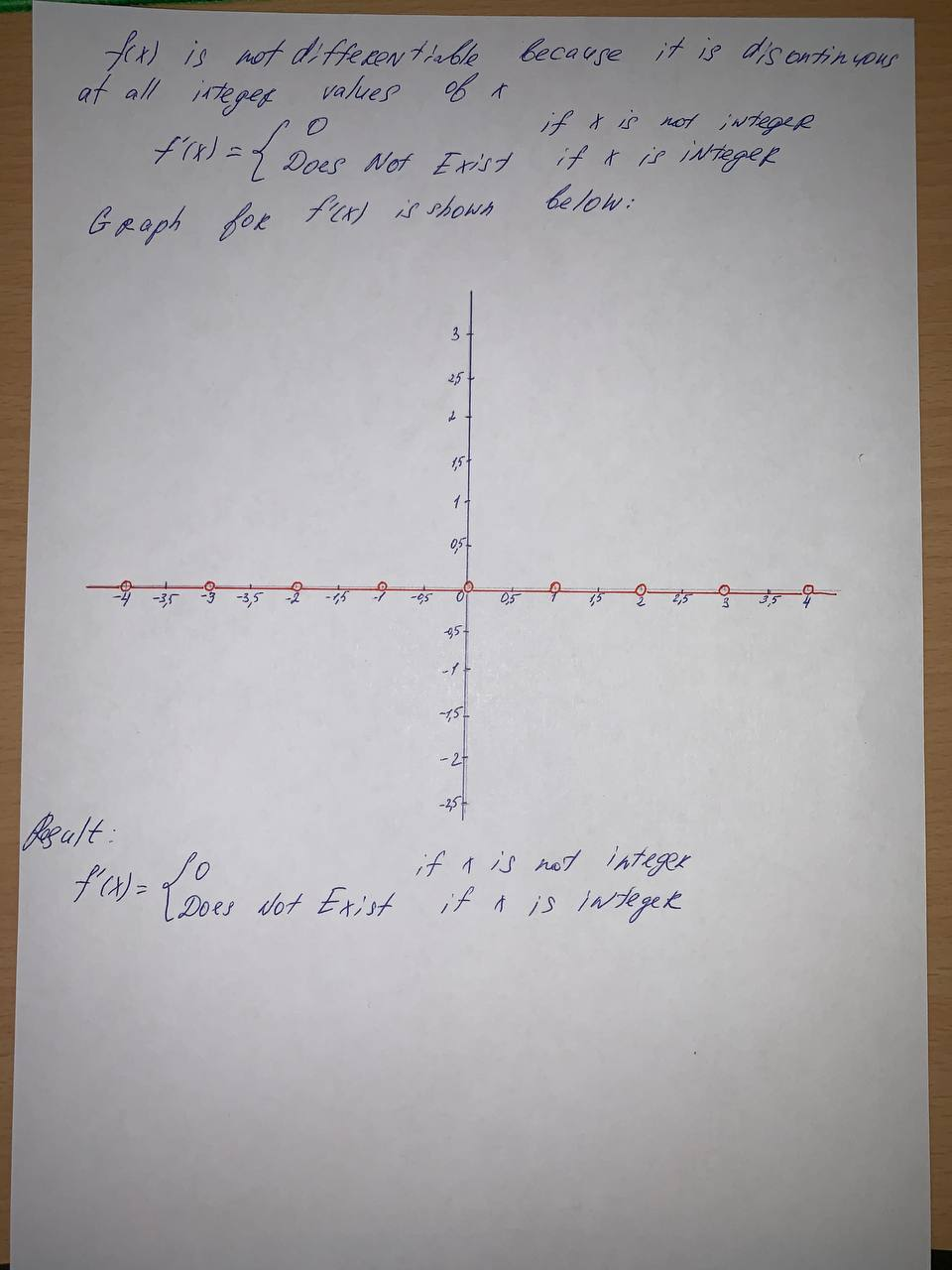Where is the greatest integer function f(x) =[[x]] not differentiable? FindBurhan Hopper 2021-08-10 Answered
Where is the greatest integer function $$\displaystyle{f{{\left({x}\right)}}}={\left[{\left[{x}\right]}\right]}$$ not differentiable?
Find a formula for f’ and sketch its graph.

• Questions are typically answered in as fast as 30 minutes

Solve your problem for the price of one coffee

• Math expert for every subject
• Pay only if we can solve itgwibdaithq

To sketch a graph you need